# Numerical integral with a singularity

Richard Hamming  gives this nice example of an integral with a mild singularity:The integrand approaches −∞ as x approaches 0 and yet the integral is finite. If we try into numerically evaluate this integral, we will either get inaccurate results or we will have to go to a lot of work.

This post will show that with a clever reformulation of the problem we use simple methods to get accurate results, or use sophisticated methods with fewer function evaluations.

As I wrote about years ago, a common technique for dealing with singularities is to add and subtract a function with the same asymptotic behavior that can be integrated by hand. Hamming does a slight variation on this, multiplying and dividing by x inside the logarithm.Here we integrated the singular part, log(x), and we are left with numerically integrating a well-behaved function, one that is smooth and bounded on the interval of integration. Because sin(x) ≈ x for small x, we can define sin(x)/x to be 1 at 0 and have a smooth function.

We’ll use NumPy’s sinc function to handle sin(x)/x properly near 0. There are two conventions for defining the sinc function, either as sin(x)/x or as sin(πx)/πx. NumPy uses the latter convention, so we define our own sinc function as follows.

import numpy as np
def mysinc(x): return np.sinc(x/np.pi)


## Trapezoid rule

Let’s use the simplest numerical method, the trapezoid rule.

We run into a problem immediately: if we chop up the interval [0, 1] in a way that puts an integration point at 0, our resulting integral will be infinite. We could replace 0 with some ε > 0, but if we do, we should try a few different values of ε to see whether our choice of ε greatly impacts our integral.

for ε in [1e-6, 1e-7, 1e-8]:
xs = np.linspace(ε, 1, 100)
integral = sum( np.log(np.sin(x)) for x in xs ) / 100


This gives us

-1.152996659484881
-1.1760261818509377
-1.1990523999312201


suggesting that the integral does indeed depend on ε. We’ll see soon that our integral evaluates to around −1.05. So our results are not accurate, and the smaller ε is, the worse our answer is. 

Now let’s evaluate the integral as Hamming suggests. We’ll use a varying number of integration points so the difference between our integral estimates will give us some idea whether we’re converging.

for N in [25, 50, 100]:
xs = np.linspace(0, 1, N)
integral = sum( np.log(mysinc(xs)) )/N
integral -= 1 # subtract the integral of log(x)
print(integral)


This gives us

-1.0579531812873197
-1.057324013010989
-1.057019035348765


The consistency between the result suggests the integral is around −1.057.

We said at the top of this article that Hamming’s reformulation would either let us get accurate results from simple methods or let us get by with fewer function evaluations using a sophisticated method. Now we’ll demonstrate the latter, using the adaptive integration algorithm quad from SciPy.

from scipy.integrate import quad

# Integrate log(sin(x)) from 0 to 1
integral, error = quad(lambda x: np.log(np.sin(x)), 0, 1)
print(integral, error)

# Integrate log(x sin(x)/x) = log(x) + log(mysinc(x)) from 0 to 1
integral, error = quad(lambda x: np.log(mysinc(x)), 0, 1)
integral -= 1
print(integral, error)


This prints

-1.0567202059915843 1.7763568394002505e-15
-1.056720205991585 6.297207865333937e-16


suggesting that both approaches are working. Both estimate their error to be near machine precision. But as we’ll see, the direct approach uses about 10 times as many function evaluations.

Let’s ask quad to return more details by adding full_output = 1 as an argument.

integral, error, info = quad(lambda x: np.log(np.sin(x)), 0, 1, full_output=1)
print(integral, error, info["neval"])

integral, error, info = quad(lambda x: np.log(mysinc(x)), 0, 1, full_output=1)
integral -= 1
print(integral, error, info["neval"])


This shows that the first integration used 231 function evaluations, but the second used only 21.

The difference in efficiency doesn’t matter when you’re evaluating an integral one time, but if you were repeatedly evaluating similar integrals inside a loop, subtracting off the singularity could make your problem run 10 times faster. Simulations involving Bayesian statistics can have such integrations in the inner loop, and so making an integration an order of magnitude faster could make the overall simulation an order of magnitude faster, reducing CPU-days to CPU-hours.

## Related posts

 Richard Hamming, Numerical Methods for Scientists and Engineers. Second edition. Dover.

 We can get better results if we let ε and 1/N go to zero at the same rate. The following code produces mediocre results, but better results than above.

for N in [10, 100, 1000]:
xs = np.linspace(1/N, 1, N)
integral = sum( np.log(np.sin(x)) for x in xs ) / N
print(integral)


This prints

-0.8577924592777062
-1.0253626377143321
-1.05243363818431


which at least seems to be getting closer to the correct result, but has bad accuracy for so many function evaluations.

# Equation of an ellipse or hyperbola through five points

Yesterday I wrote about the equation of a circle through three points. This post will be similar, looking at the equation of an ellipse or hyperbola through five points. As with the earlier post, we can write down an elegant equation right away. Making the equation practical will take more work.

The equation of a general conic section through five points## Direct but inefficient solution

The most direct (though not the most efficient) way to turn this into an equationis to expand the determinant by minors of the first row.## More efficient solution

It would be much more efficient to solve a system of equations for the coefficients. Since the determinant at the top of the page is zero, the columns are linearly dependent, and some linear combination of those columns equals the zero vector. In fact, the coefficients of such a linear combination are the coefficients A through F that we’re looking for.

We have an unneeded degree of freedom since any multiple of the coefficients is valid. If we divide every coefficient by A, then the new leading coefficient is 1. This gives us five equations in five unknowns.## Python example

The following code will generate five points and set up the system of equations Ms = b whose solution s will give our coefficients.

    import numpy as np

np.random.seed(20230619)

M = np.zeros((5,5))
b = np.zeros(5)
for i in range(5):
x = np.random.random()
y = np.random.random()
M[i, 0] = y**2
M[i, 1] = x*y
M[i, 2] = x
M[i, 3] = y
M[i, 4] = 1
b[i] = -x*x


Now we solve for the coefficients.

    s = np.linalg.solve(M, b)
A, B, C, D, E, F = 1, s, s, s, s, s


Next we verify that the points we generated indeed lie on the conic section whose equation we just found.

    for i in range(5):
x = M[i, 2]
y = M[i, 3]
assert( abs(A*x*x + B*y*y + C*x*y + D*x + E*y + F) < 1e-10 )


## Ellipse or hyperbola?

It turns out that the five points generated above lie on a hyperbola.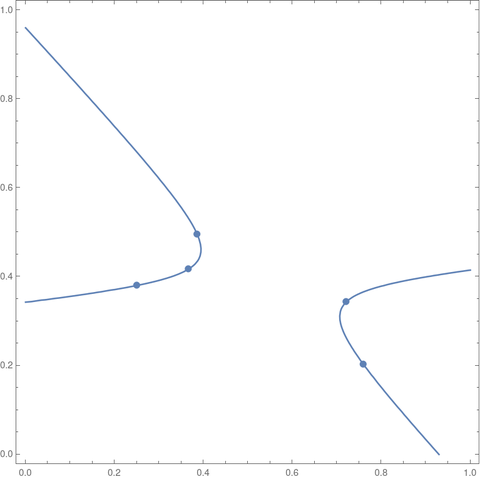If we had set the random number generator seed to 20230620 we would have gotten an ellipse.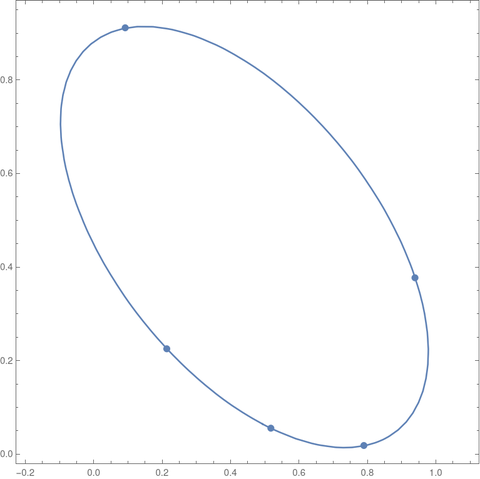When we generate points at random, as we did above, we will almost certainly get an ellipse or a hyperbola. Since we’re generating pseudorandom numbers, there is some extremely small chance the generated points would lie on a line or on a parabola. In theory, this almost never happens, in the technical sense of “almost never.”

If 4ABC² is positive we have a an ellipse. If it is negative we have a hyperbola. If it is zero, we have a parabola.

# AM over GM

Suppose you take the arithmetic mean and the geometric mean of the first n integers. The ratio of these two means converges to e/2 as n grows . In symbols,Now suppose we wanted to visualize the convergence by plotting the expression on the left side for a sequence of ns.

First let’s let n run from 1 to 100.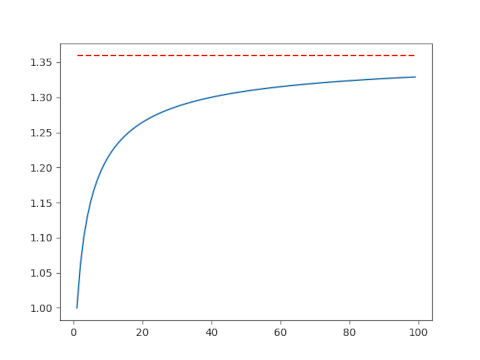This isn’t very convincing. Maybe the convergence is just slow, or maybe it’s not actually converging to e/2. Let’s make another plot including larger values of n. This will require a little additional work.

## Avoiding overflow

Here’s the code that made the plot above.

    import matplotlib.pyplot as plt
import numpy as np
from scipy.special import factorial

AM = lambda n: (n+1)/2
GM = lambda n: factorial(n)**(1/n)
n = np.arange(1, 100)
plt.plot(n, AM(n)/GM(n))
plt.plot(n, 0*n + np.exp(1)/2, 'r--')


This works for n up to 170, but it fails when n = 171 because at that point factorial overflows.

This is a very common situation. We ultimately want to compute a fairly small number, but we encounter extremely large numbers in an intermediate step.

We need to avoid directly calculating n! before taking the nth root. The way to do this is to work with logarithms. Now n! = Γ(n+1) and SciPy has a function for computing the log of the gamma function directly without first computing the gamma function, thus avoiding overflow.

We replace GM above with GM2 below:

    GM2 = lambda n: np.exp(gammaln(n+1)/n)

Now we compute the geometric mean of the first n natural numbers for very large values of n. We only need n = 1000 to see convergence, but the code could handle much larger values of n without overflowing.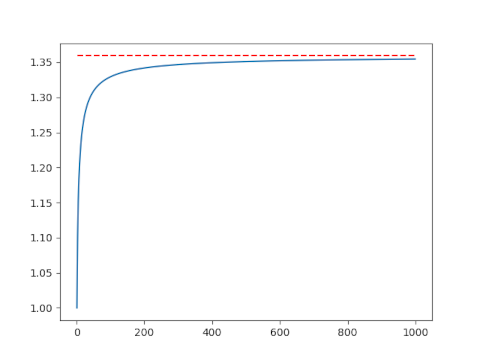## Related posts

 Richard P. Kubelka. Means to an End. Mathematics Magazine, Vol. 74, No. 2 (April 2001), pp. 141–142

# Solve for Jacobi sn parameter to have given period(s)

Here’s a calculation that I’ve had to do a few times. I’m writing it up here for my future reference and for the benefit of anybody else who needs to do the same calculation.

The Jacobi sn function is doubly periodic: it has one period as you move along the real axis and another period as you move along the imaginary axis. Its values are determined by a fundamental rectangle, and here we solve for a parameter 0 ≤ m < 1 that yields a desired length, or width, or aspect ratio for this rectangle.

You cannot specify both the length and width of the fundamental rectangle independently: you only have one degree of freedom. You can specify its length, or width, or aspect ratio.

This post focuses on sn, then explains how to modify the results for the other Jacobi elliptic functions—cn, dn, sc, etc.—at the end.

## Given period

A parameter value m corresponds to a horizontal period of 4K(m) and a vertical period of 2K(1-m) where K is the complete elliptic integral of the first kind.

K(m) is an increasing function from [0, 1) to [π/2, ∞). So the minimum horizontal period is 2π and the minimum vertical period is π.

## Given aspect ratio

The ratio of the horizontal period to the vertical period is given by

r(m) = 4K(m) / 2K(1-m) = 2K(m)/K(1-m).

The function r is an increasing function from [0, 1) to [0, ∞), so any aspect ratio is possible. This is important, for example, in conformal mapping. You cannot specify the length and width of the fundamental period independently, but you can solve for the parameter m that gives the aspect ratio you want, then follow it by a dilation to then get the length and width you want.

## Numerical computation

If you need to find m efficiently or to high precision, see . The code here simple and good enough for my purposes, and hopefully good enough for yours.

We need to either invert K or r, both of which are monotone functions, and so we can use the bisection method to find the value of m we’re after. The bisection method is not the most efficient, but it’s simple and very robust.

## Python code

    from numpy import pi, linspace
from scipy.special import ellipk, ellipj
from scipy.optimize import bisect

def invert_ellipk(K):
"Given K, find m such that K(m) = K."
assert(K >= pi/2)
if K == pi/2:
return 0

# Find a bracket [a, b] for m
a = 0
b = 0.5
y = ellipk(b)
while y < K:
b = 0.5*(b + 1)
y = ellipk(b)
return bisect(lambda m: ellipk(m)-K, a, b)

def invert_horizontal_period(T):
"Find parameter m such that sn(z, m) has real period T."
return invert_ellipk(T/4)

def invert_vertical_period(T):
"Find parameter m such that sn(z, m) has imaginary period T."
return invert_ellipk(T/2)

def invert_r(r):
"Find parameter m such that the fundamental rectangle of sn(z, m) has aspect ratio r."

ratio = lambda m: 2*ellipk(m)/ellipk(1-m)

if r == 2:
return 1/2

a, b = 0.5, 0.5
while ratio(b) < r: b = 0.5*(1 + b) while ratio(a) > r:
a /= 2
return bisect(lambda m: r - ratio(m), a, b)


To test the code, let’s find m such that sn(z, m) has period 5 along the real axis.

    import matplotlib.pyplot as plt

T = 16
m = invert_horizontal_period(T)
print(4*ellipk(m))
assert(abs(4*ellipk(m) - T) < 1e-8)
x = linspace(0, 2*T, 200)
plt.plot(x, sn(x, m))


This produces a plot that does have period 16.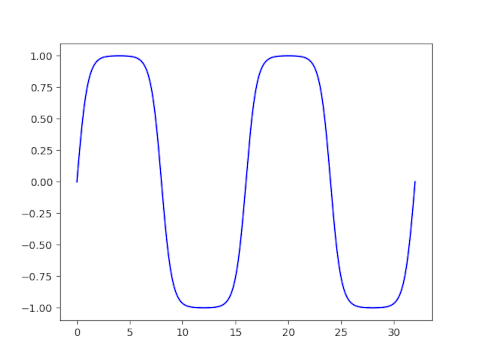## Other Jacobi elliptic functions

There are 12 Jacobi elliptic functions, denoted with all pairs of {s, c, d, n} such that the two letters are different. The periods of the functions fall into three groups.

The functions sn, ns, cd, and dc all have real period 4K(m) and imaginary period 2K(1-m).

The functions cn, nc, ds, and sd all have real period 4K(m) and imaginary period 4K(1-m).

The functions cs, sc, dn, and nd all have real period 2K(m) and imaginary period 4K(1-m).

You can find plots of fundamental rectangles of these 12 functions here.

 Toshio Fukushima. Numerical computation of inverse complete elliptic integrals of the first and second kinds. Journal of Computational and Applied Mathematics. 249 (2013) 37–50.

# Logarithmic spiral

I’ve seen an image similar to the following many times, but I don’t recall any source going into detail regarding how the spiral is constructed. This post will do just that.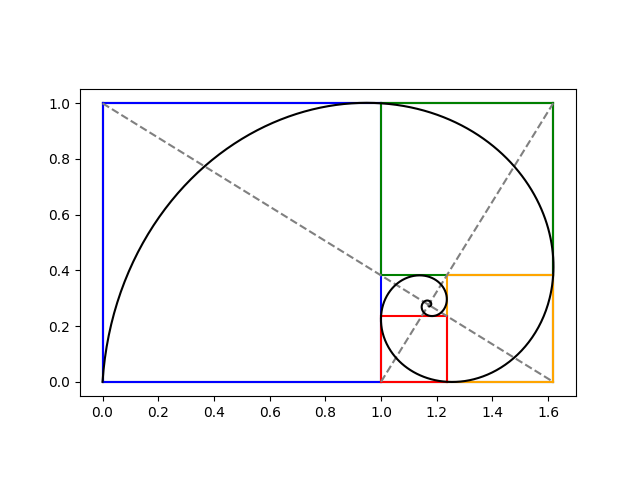The previous post constructed iterated golden rectangles. We start with a golden rectangle and imagine chopping of first the blue, then the green, then the orange, and then the red square. We could continue this process on the inner most rectangle, over and over.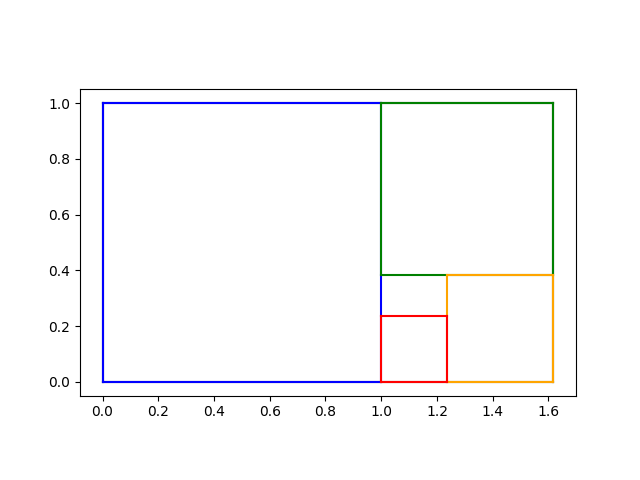At this point, many sources would say “Hey look! We can draw a spiral that goes through two corners of each square” without explicitly stating an equation for the spiral. We’ll find spiral, making everything explicit.

In the previous post we showed how to find the limit of the iterative process at the intersection of the two diagonal lines below.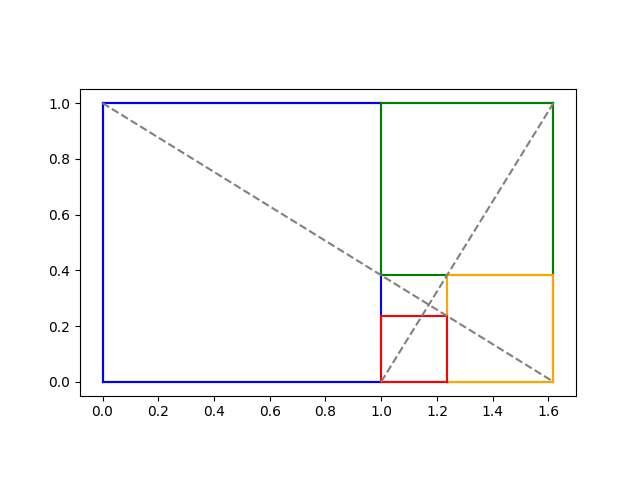We showed that the intersection is at

x0 = (2φ + 1)/(φ + 2)

and

y0 = 1/(φ + 2).

## Logarithmic spirals

A logarithmic spiral centered at the origin has the polar equation

r(θ) = a exp(kθ)

for some constants a and k. Our spiral will be such a spiral shifted to center at (x0y0).

Imagine a spiral going from the lower left to the top left of the blue square, then to the top left of the green square, then to the top right of the orange square etc., passing through two corners of every square. Every time θ goes through a quarter turn, i.e. π/2 radians, our rectangles get smaller by a factor of φ. We can use this to solve for k because

a exp(k(θ + π/2)) = φ a exp()

and so

exp(kπ/2) = φ

and

k = 2log(φ) / π.

To summarize what we know so far, if we shift our spiral to the origin, it has equation in polar form

r(θ) = a exp(kθ)

where we now know k but don’t yet know a.

## Finding θ and a

To get our actual spiral, we have to shift the origin to (x0, y0) which we have calculated above. Converting from polar to rectangular coordinates so we can do the shift, we have

x(θ) = x0 + a exp(kθ) cos(θ)

y(θ) = y0 + a exp(kθ) sin(θ)

We can find the parameter a by knowing that our spiral passes through the origin, the bottom left corner in the plots above. At what value of θ does the curve go through the origin? We have a choice; values of θ that differ by multiples of 2π will give different values of a.

The angle θ is the angle made by the line connecting (0, 0) to (x0, y0). We can find θ via

θ0 = atan2(-y0, –x0).

Here’s Python code to find θ0:

    import numpy as np

phi = (1 + 5**0.5)/2
y0 = 1/(2+phi)
x0 = (2*phi + 1)*y0
theta0 = np.arctan2(-y0, -x0)


Then we solve for a from the equation x0) = 0.

    k = 2*np.log(phi)/np.pi
a = -x0 / (np.exp(k*theta0)*np.cos(theta0)))

## Plots

Now we have all the parameters we need to define our logarithmic spiral. The following code draws our logarithmic spiral on top of the plot of the rectangles.

    t = np.linspace(-20, theta0, 1000)
def x(t): return x0 + a*np.exp(k*t)*np.cos(t)
def y(t): return y0 + a*np.exp(k*t)*np.sin(t)
plt.plot(x(t), y(t), "k-")


The result is the image at the top of the post.

# Find log normal parameters for given mean and variance

Earlier today I needed to solve for log normal parameters that yield a given mean and variance. I’m going to save the calculation here in case I needed in the future or in case a reader needs it. The derivation is simple, but in the heat of the moment I’d rather look it up and keep going with my train of thought.

NB: The parameters μ and σ² of a log normal distribution are not the mean and variance of the distribution; they are the mean and variance of its log.

If m is the mean and v is the variance thenNotice that the square of the m term matches the second part of the v term.

Thenand soand once you have σ² you can find μ byHere’s Python code to implement the above.

    from numpy immport log
def solve_for_log_normal_parameters(mean, variance):
sigma2 = log(variance/mean**2 + 1)
mu = log(mean) - sigma2/2
return (mu, sigma2)


And here’s a little test code for the code above.

    mean = 3.4
variance = 5.6

mu, sigma2 = solve_for_log_normal_parameters(mean, variance)

X = lognorm(scale=exp(mu), s=sigma2**0.5)
assert(abs(mean - X.mean()) < 1e-10)
assert(abs(variance - X.var()) < 1e-10)


# Finding Lagrange points L1 and L2

The James Webb Space Telescope (JWST) is on its way to the Lagrange point L2 of the Sun-Earth system. Objects in this location will orbit the Sun at a fixed distance from Earth.

There are five Lagrange points, L1 through L5. The points L1, L2, and L3 are unstable, and points L4 and L5 are stable. Since L2 is unstable, it will have to adjust its orbit occasionally to stay at L2.

The Lagrange points L1 and L2 are nearly equally distant from Earth, with L1 between the Earth and Sun, and L2 on the opposite side of Earth from the Sun.

The equations for the distance r from L1 and L2 to Earth are very similar and can be combined into one equation:The equation for L1 corresponds to taking ± as – and the equation for L2 corresponds to taking ± as +.

In the equation above, R is the distance between the Sun and Earth, and M1 and M2 are the mass of the Sun and Earth respectively. (This is not going to be too precise since the distance between the Sun and Earth is not constant. We’ll use the mean distance for R.)

For both L1 and L2 we haveLet’s use Newton’s method to solve for the distances to L1 and L2, and see how they compare to the approximation above.

    from scipy.optimize import newton

M1 = 1.988e30 # kg
M2 = 5.972e24 # kg
R  = 1.471e8  # km

approx = R*(M2/(3*M1))**(1/3)

def f(r, sign):
M = M1 + M2
ret = M1/(R + sign*r)**2 + sign*M2/r**2
ret -= (R*M1/M + sign*r)*M/R**3
return ret

L1 = newton(lambda r: f(r, -1), approx)
L2 = newton(lambda r: f(r,  1), approx)

print("L1       = ", L1)
print("approx   = ", approx)
print("L2       = ", L2)
print("L1 error = ", (approx - L1)/L1)
print("L2 error = ", (L2 - approx)/L2)


This prints the following.


L1       = 1467000
approx   = 1472000
L2       = 1477000
L1 error = 0.3357%
L2 error = 0.3312%


So L2 is slightly further away than L1. The approximate distance under-estimates L2 and over-estimates L1 both by about 0.33% .

L1 and L2 are about 4 times further away than the moon.

## Related posts

 The approximation for r makes an excellent starting point. When I set the relative error target to 1e-5, Newton’s method converged in four iterations.

    full = newton(lambda r: f(r, 1), approx, tol=1e-5, full_output=True)
print(full)


# Numbering the branches of the Lambert W function

The previous post used the Lambert W function to solve an equation that came up in counting partitions of an integer. The first solution found didn’t make sense in context, but another solution, one on a different branch, did. The default branch k = 0 wasn’t what we were after, but the branch k = -1 was.

## Branches 0 and -1

The branch corresponding to k = 0 is the principal branch. In mathematical notation, the k is usually a subscript, i.e W0(z) is the principal branch of the Lambert W function. For real z ≥ -1/e it returns a real value. It’s often what you want, and that’s why it’s the default in software implementations such as SciPy and Mathematica. More on that below.

The only other branch that returns real values for real inputs is W-1 which returns real values for -1/ez < 0.

If you’re working strictly with real numbers, you only need to be concerned with branches 0 and -1. If branch 0 doesn’t give you what you expect, try branch -1, if your argument is negative.

## SciPy and Mathematica

SciPy implements Wk with lambertw(z). The parameter k defaults to 0.

The Mathematica function ProductLog[z] implements W0(z), and ProductLog[k, z] implements Wk.

Note that Mathematica and Python list their arguments in opposite orders.

## Branch numbering

The branches are numbered in order of their imaginary parts. That is, The imaginary part of Wk (z) is an increasing function of k. For example, here is a list of the numerical values of the imaginary parts of Wk (1) for k running from -3 to 3.

    Table[N[Im[ProductLog[k, 1]]], {k, -3, 3}]
{-17.1135, -10.7763, -4.37519, 0., 4.37519, 10.7763, 17.1135}


Note the zero in the middle because W0(1) is real.

## Recovering k

Given z and a value Wk (z) with k unknown, you can determine k withwith the exception that if the expression above is 0 and -1/ez < 0 then k = -1. See .

For example, let z = 1 + 2i and w = W3 (z).

    z = 1 + 2 I
w = ProductLog[3, z]


Now pretend you don’t know how w was computed. When you compute

    N[(w + Log[w] - Log[z])/(2 Pi I)]

the result is 3.

## Partition example

The discussion of the W function here grew out of a problem with partitions. Specifically, we wanted to solve for n such thatis approximately a trillion. We found in the previous post that solutions of the equationare given byOur partition problem corresponds to a = 1/√48, b = -1, c = π √(2/3), and d = 1/2. We found that the solution we were after came from the k = -1 branch.

    N[( (b/(c d)) ProductLog[-1, (x/a)^(d/b) c d /b])^(1/d)]
183.852


Even though our final value was real, the intermediate values were not. The invocation of W-1 in the middle returns a complex value:

    N[ProductLog[-1, ((x/a)^(d/b) c d /b)^(1/d)]]
-32.5568 - 3.24081 I


We weren’t careful about specifying ranges and branch cuts, but just sorta bulldozed our way to a solution. But it worked: it’s easy to verify that 183.852 is the solution we were after.

***

 Unwinding the branches of the Lambert W function by Jeffrey, Hare, and Corless. The Mathematical Scientist 21, 1&ndash;7 (1996)

# Solving equations with Lambert’s W function

In the previous post we wanted to find a value of n such that f(n) = 1012 whereand we took a rough guess n = 200. Turns out f(200) ≈ 4 × 1012 and that was good enough for our purposes. But what if we wanted to solve f(n) = x accurately?

We will work our way up to solving f(n) = 1012 exactly using the Lambert W function.

## Lambert’s W function

If you can solve one equation involving exponential functions you can bootstrap your solution solve a lot more equations.

The Lambert W function is defined to be the function W(x) that maps each x to a solution of the equation

w exp(w) = x.

This function is implemented Python under scipy.special.lambertw. Let’s see how we could use it solve similar equations to the one that define it.

## Basic bootstrapping

Given a constant c we can solve

cw exp(w) = x

simply by solving

w exp(w) = x/c,

which says w = W(x/c).

And we can solve

w exp(cw) = x

by setting y = cw and solving

y exp(y) = cx.

and so cw = W(cx) and w = W(cx)/c.

Combining the two approaches above shows that the solution to

aw exp(bw) = x

is

w = W(bx/a) / b.

We can solve

exp(w) / w = x

by taking the reciprocal of both sides and applying the result above with a = 1 and b = -1.

More generally, we can solve

wc exp(w) = x

by raising both sides to the power 1/c; the reciprocal the special case c = 1.

Similarly, we can solve

w exp(wc) = x

by setting y = wc , changing the problem to

y-1/c exp(y) = x.

## Putting it all together

We’ve now found how to deal with constants and exponents on w, inside and outside the exponential function. We now have all the elements to solve our original problem.

We can solve the general equationwithThe equation at the top of the post corresponds to a = 1/√48, b = -1, c = π √(2/3), and d = 1/2.

We can code this up in Python as follows.

    from scipy.special import lambertw

def soln(a, b, c, d, x):
t = (x/a)**(d/b) *c*d/b
return (lambertw(t)*b/(c*d))**(1/d)


We can verify that our solution really is a solution by running it though

    from numpy import exp, pi

def f(a, b, c, d, w):
return a*w**b * exp(c*w**d)


to make sure we get our x back.

When we run

    a = 0.25*3**-0.5
b = -1
c = pi*(2/3)**0.5
d = 0.5
x = 10**12

w = soln(a, b, c, d, x)
print(f(a, b, c, d, w))


we do indeed get x back.

    a = 0.25*3**-0.5
b = -1
c = pi*(2/3)**0.5
d = 0.5

w = soln(a, b, c, d, 10)
print(f(a, b, c, d, w))


When we take a look at the solution w, we get 1.443377079584187e-13. In other words, we get a number near zero. But our initial guess was w = 200. What went wrong?

Nothing went wrong. We got a solution to our equation. It just wasn’t the solution we expected.

The Lambert W function has multiple branches when viewed as a function on complex numbers. It turns out the solution we were expecting is on the branch SciPy denotes with k = -1. If we add this to the call to lambertw above we get the solution 183.85249773880142 which is more in line with what we expected.

# Lambert W strikes again

I was studying a statistics paper the other day in which the author said to solve

t log( 1 + n/t ) = k

for t as part of an algorithm. Assume 0 < k < n.

## Is this well posed?

First of all, can this equation be solved for t? Second, if there is a solution, is it unique?

It’s not hard to show that as a function of t, the left side approaches 0 as t approaches 0, and it approaches n as t goes to infinity. So there is a solution for all k between 0 and n. The restriction on k is necessary since the left side cannot exceed n.

With a little more work one can show that the derivative is always positive, so the left side is a monotonically increasing function, and so the solution given each value of k is unique.

## Analytic solution

Now if we fix n, we can think of the equation above as defining t as a function of k. Can we solve for t exactly? I suspected that there might be a solution in terms of the Lambert W function because if you divide by t and exponentiate, you get an equation that smells like the equation

z = w exp(w)

defining the function W(z). It turns out this is indeed the case.

    Solve[t Log[1 + n/t] ==  k, t]

we getGreat! There’s a closed-form solution, if you accept using the W function as being closed form.

## Problems with the solution

I found the solution in Mathematica, but I tested it in Python to make sure both platforms define W the same way.

    from numpy import log, exp
from scipy.special import lambertw

def f(t, n):
return t*log(1 + n/t)

def g(k, n):
r = k/n
return -k/(lambertw(-r*exp(-r)) + r)

n, k = 10, 8
t = g(k, n)
print(f(t, n))


This should print k, so it prints 8, right? No, it prints 10.

What’s up with that?

If we look back at the equation for t above, we see that the W function is being evaluated at x exp(x) where x = –k/n, so we should get –k/n back since W(x exp(x)) = x by definition. But that means our denominator is zero, and so the equation doesn’t make sense!

Things are getting worse. At first we had a wrong value, but at least it was finite!

The problem is not a difference between Mathematica and Python.

## Resolution

The problem is we’ve glossed over a branch cut of the W function. To make a long story short, we were using the principal branch of the W function, but we should have used a different branch.

Let’s go back to where I asked Mathematica

    Solve[t Log[1 + n/t] == k, t]

I ran the solution through TeXForm to get the TeX code that produced the image for the solution equation. I made a few aesthetic changes to the TeX, but it was essentially Mathematica’s output.

Without the TeXForm, Mathematica’s solution was in terms of ProductLog, not in terms of W; the TeXForm function turned ProductLog into W. If you look up ProductLog, it will tell you

ProductLog[z] gives the principal solution for w in z = wew.

The principal solution. So we should be on the alert for difficulties with branches. There are two real solutions to z = wew for some values of z, and we have to choose the right one. For example, if z = -0.1, the w could be -0.1118 or -3.5772.

Mathematica gave me the wrong branch. But to be fair, it did try to warn me.

Solve::ifun: Inverse functions are being used by Solve, so some solutions may not be found; use Reduce for complete solution information.

The solution is to use the -1 branch. In Mathematica’s notation, the branch comes before the argument. In SciPy, the branch comes second. So to fix the code above, we need to change

    lambertw(-r*exp(-r))

to

   lambertw(-r*exp(-r), -1)

and then the code will be correct.

If x is negative, and we use the -1 branch of W, then

W-1(x exp(x)) ≠ x

and so we’re not dividing by zero in our solution.